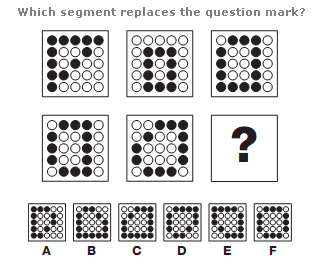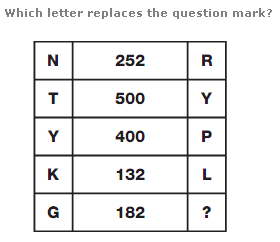# Puzzles - Logical puzzles

### Exercise :: Logical puzzlesAnswer : Doughnut Explanation : The Doughnut = 4, the Eclair = 6 and the Bun = 7.

View Answer & ExplanationAnswer : F Explanation : In each diagram, the black circles join together to make straight sided polygons. Working from left to right, top row then bottom row, the number of sides in each polygon increases by 1 each time, from 3 to 8.

View Answer & ExplanationAnswer : Z Explanation : In each row, multiply the numerical values of the left and right hand letters, putting the result in the centre.

View Answer & Explanation

#### Current Affairs 2019

Interview Questions and Answers